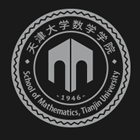﻿Strictly positive semidefinite tensor and monotone stochastic tensor complementarity problem-天津大学数学学院

# Strictly positive semidefinite tensor and monotone stochastic tensor complementarity problem

2019-11-05 14:09

In this paper, we first introduce a new class of structured tensors, named strictly positive semidefinite tensors, and show that a strictly positive semidefinite tensor is not an \$R_0\$ tensor. We focus on studying the stochastic tensor complementarity problem (STCP), where the expectation of the involved tensor is a strictly positive semidefinite tensor. We denote such an STCP as a monotone STCP. Based on three popular NCP functionsminfunction,FBfunction and thepenalized FBfunction, as well as the special structure of the monotone STCP, we introduce three new NCP functions and establish the expected residual minimization (ERM) formulation of the monotone STCP. We show that the solution set of the ERM problem is nonempty and bounded if the solution set of the expected value (EV) formulation for such an STCP is nonempty and bounded. Moreover, an approximate regularized model is proposed to weaken the conditions for non-emptiness and boundedness of the solution set of the ERM problem in practice. Numerical results indicate that the performance of the ERM method is better than that of the EV method.### 联系我们